# sklearn.naive_bayes.GaussianNB¶

class sklearn.naive_bayes.GaussianNB(*, priors=None, var_smoothing=1e-09)[source]

Gaussian Naive Bayes (GaussianNB).

Can perform online updates to model parameters via partial_fit. For details on algorithm used to update feature means and variance online, see Stanford CS tech report STAN-CS-79-773 by Chan, Golub, and LeVeque:

Read more in the User Guide.

Parameters:
priorsarray-like of shape (n_classes,)

Prior probabilities of the classes. If specified, the priors are not adjusted according to the data.

var_smoothingfloat, default=1e-9

Portion of the largest variance of all features that is added to variances for calculation stability.

New in version 0.20.

Attributes:
class_count_ndarray of shape (n_classes,)

number of training samples observed in each class.

class_prior_ndarray of shape (n_classes,)

probability of each class.

classes_ndarray of shape (n_classes,)

class labels known to the classifier.

epsilon_float

n_features_in_int

Number of features seen during fit.

New in version 0.24.

feature_names_in_ndarray of shape (n_features_in_,)

Names of features seen during fit. Defined only when X has feature names that are all strings.

New in version 1.0.

sigma_ndarray of shape (n_classes, n_features)

DEPRECATED: Attribute sigma_ was deprecated in 1.0 and will be removed in1.2.

var_ndarray of shape (n_classes, n_features)

Variance of each feature per class.

New in version 1.0.

theta_ndarray of shape (n_classes, n_features)

mean of each feature per class.

BernoulliNB

Naive Bayes classifier for multivariate Bernoulli models.

CategoricalNB

Naive Bayes classifier for categorical features.

ComplementNB

Complement Naive Bayes classifier.

MultinomialNB

Naive Bayes classifier for multinomial models.

Examples

>>> import numpy as np
>>> X = np.array([[-1, -1], [-2, -1], [-3, -2], [1, 1], [2, 1], [3, 2]])
>>> Y = np.array([1, 1, 1, 2, 2, 2])
>>> from sklearn.naive_bayes import GaussianNB
>>> clf = GaussianNB()
>>> clf.fit(X, Y)
GaussianNB()
>>> print(clf.predict([[-0.8, -1]]))

>>> clf_pf = GaussianNB()
>>> clf_pf.partial_fit(X, Y, np.unique(Y))
GaussianNB()
>>> print(clf_pf.predict([[-0.8, -1]]))



Methods

 fit(X, y[, sample_weight]) Fit Gaussian Naive Bayes according to X, y. get_params([deep]) Get parameters for this estimator. partial_fit(X, y[, classes, sample_weight]) Incremental fit on a batch of samples. Perform classification on an array of test vectors X. Return log-probability estimates for the test vector X. Return probability estimates for the test vector X. score(X, y[, sample_weight]) Return the mean accuracy on the given test data and labels. set_params(**params) Set the parameters of this estimator.
fit(X, y, sample_weight=None)[source]

Fit Gaussian Naive Bayes according to X, y.

Parameters:
Xarray-like of shape (n_samples, n_features)

Training vectors, where n_samples is the number of samples and n_features is the number of features.

yarray-like of shape (n_samples,)

Target values.

sample_weightarray-like of shape (n_samples,), default=None

Weights applied to individual samples (1. for unweighted).

New in version 0.17: Gaussian Naive Bayes supports fitting with sample_weight.

Returns:
selfobject

Returns the instance itself.

get_params(deep=True)[source]

Get parameters for this estimator.

Parameters:
deepbool, default=True

If True, will return the parameters for this estimator and contained subobjects that are estimators.

Returns:
paramsdict

Parameter names mapped to their values.

partial_fit(X, y, classes=None, sample_weight=None)[source]

Incremental fit on a batch of samples.

This method is expected to be called several times consecutively on different chunks of a dataset so as to implement out-of-core or online learning.

This is especially useful when the whole dataset is too big to fit in memory at once.

This method has some performance and numerical stability overhead, hence it is better to call partial_fit on chunks of data that are as large as possible (as long as fitting in the memory budget) to hide the overhead.

Parameters:
Xarray-like of shape (n_samples, n_features)

Training vectors, where n_samples is the number of samples and n_features is the number of features.

yarray-like of shape (n_samples,)

Target values.

classesarray-like of shape (n_classes,), default=None

List of all the classes that can possibly appear in the y vector.

Must be provided at the first call to partial_fit, can be omitted in subsequent calls.

sample_weightarray-like of shape (n_samples,), default=None

Weights applied to individual samples (1. for unweighted).

New in version 0.17.

Returns:
selfobject

Returns the instance itself.

predict(X)[source]

Perform classification on an array of test vectors X.

Parameters:
Xarray-like of shape (n_samples, n_features)

The input samples.

Returns:
Cndarray of shape (n_samples,)

Predicted target values for X.

predict_log_proba(X)[source]

Return log-probability estimates for the test vector X.

Parameters:
Xarray-like of shape (n_samples, n_features)

The input samples.

Returns:
Carray-like of shape (n_samples, n_classes)

Returns the log-probability of the samples for each class in the model. The columns correspond to the classes in sorted order, as they appear in the attribute classes_.

predict_proba(X)[source]

Return probability estimates for the test vector X.

Parameters:
Xarray-like of shape (n_samples, n_features)

The input samples.

Returns:
Carray-like of shape (n_samples, n_classes)

Returns the probability of the samples for each class in the model. The columns correspond to the classes in sorted order, as they appear in the attribute classes_.

score(X, y, sample_weight=None)[source]

Return the mean accuracy on the given test data and labels.

In multi-label classification, this is the subset accuracy which is a harsh metric since you require for each sample that each label set be correctly predicted.

Parameters:
Xarray-like of shape (n_samples, n_features)

Test samples.

yarray-like of shape (n_samples,) or (n_samples, n_outputs)

True labels for X.

sample_weightarray-like of shape (n_samples,), default=None

Sample weights.

Returns:
scorefloat

Mean accuracy of self.predict(X) wrt. y.

set_params(**params)[source]

Set the parameters of this estimator.

The method works on simple estimators as well as on nested objects (such as Pipeline). The latter have parameters of the form <component>__<parameter> so that it’s possible to update each component of a nested object.

Parameters:
**paramsdict

Estimator parameters.

Returns:
selfestimator instance

Estimator instance.

property sigma_

DEPRECATED: Attribute sigma_ was deprecated in 1.0 and will be removed in1.2. Use var_ instead.

## Examples using sklearn.naive_bayes.GaussianNB¶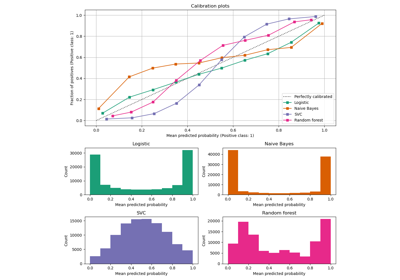Comparison of Calibration of Classifiers

Comparison of Calibration of Classifiers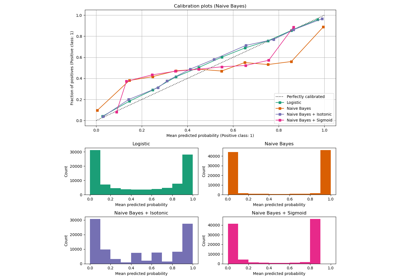Probability Calibration curves

Probability Calibration curves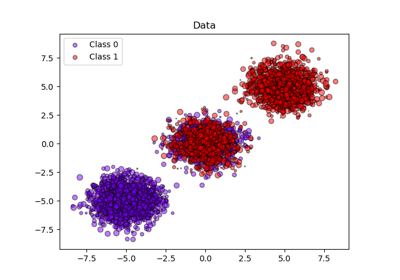Probability calibration of classifiers

Probability calibration of classifiers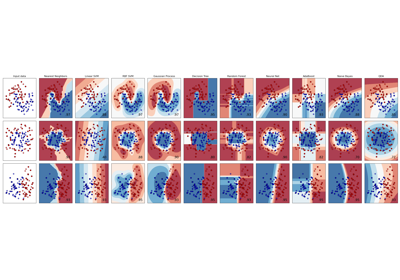Classifier comparison

Classifier comparison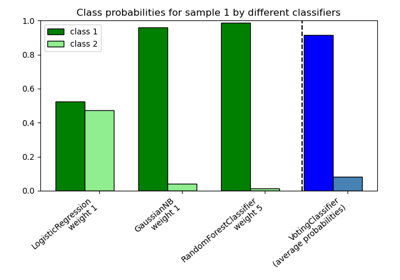Plot class probabilities calculated by the VotingClassifier

Plot class probabilities calculated by the VotingClassifier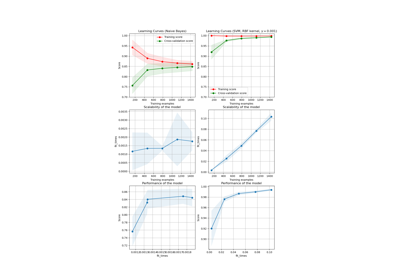Plotting Learning Curves

Plotting Learning Curves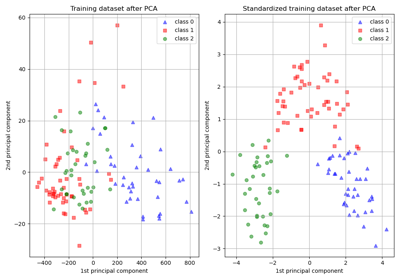Importance of Feature Scaling

Importance of Feature Scaling